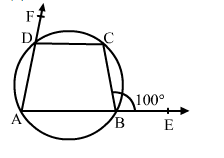# In the given figure, sides AB and AD of quad. ABCD are produced to E and F respectively. If ∠CBE = 100°, then ∠CDE = ?

Question:

In the given figure, sides AB and AD of quad. ABCD are produced to E and F respectively. If CBE = 100°, then ∠CDE = ?
(a) 100°

(b) 80°
(c) 130°
(d) 90°Solution:

(b) 80°
In a cyclic quadrilateral ABCD, we have:
Interior opposite angle, ∠ADC = exterior ∠CBE = 100°
∴ ∠CDF = (180° - ∠ADC) = (180° - 100°) = 80°   (Linear pair)